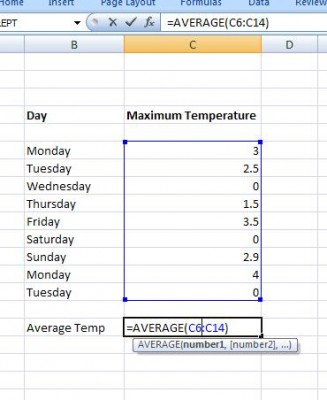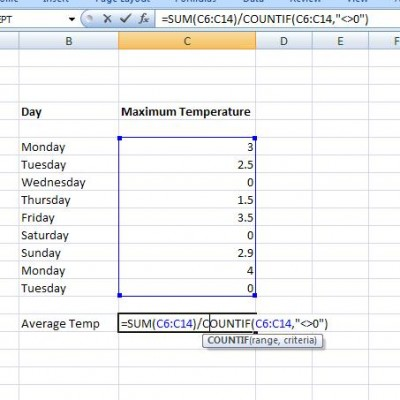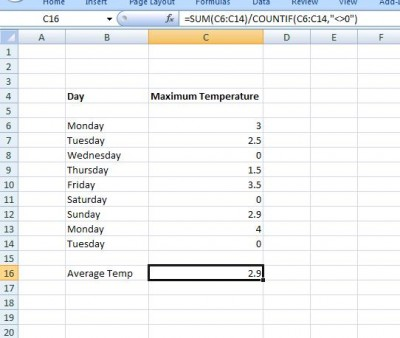# Averaging Non-Zero Values

It is quite easy to work out the average of a series of numbers:We just use the average function in Excel. However what happens if we want the average of only the NON – ZERO Values.  The function COUNTIF will count up the cells in a range that meet a criteria:

COUNTIF(Range, Criteria)
The criteria must be placed in quotes –so if our criteria is greater than 0 we need the phrase “>0”. In this case our criteria is not equal to zero.  Remembering that the average of  a series of numbers is their total divided by the total number we have:Where the “<>0” allows us to count up the non zero values in the range:### Excel Practice Worksheet

Practice Excel functions and formulas with our 100% free practice worksheets!